# Resources tagged with: Polyhedra

Filter by: Content type:
Age range:
Challenge level:

### There are 24 results

Broad Topics > 3D Geometry, Shape and Space > Polyhedra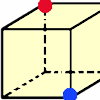### Redblue

##### Age 7 to 11 Challenge Level:

Investigate the number of paths you can take from one vertex to another in these 3D shapes. Is it possible to take an odd number and an even number of paths to the same vertex?### Icosian Game

##### Age 11 to 14 Challenge Level:

This problem is about investigating whether it is possible to start at one vertex of a platonic solid and visit every other vertex once only returning to the vertex you started at.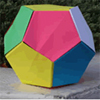### Dodecamagic

##### Age 7 to 11 Challenge Level:

Here you see the front and back views of a dodecahedron. Each vertex has been numbered so that the numbers around each pentagonal face add up to 65. Can you find all the missing numbers?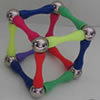### Magnetic Personality

##### Age 7 to 16 Challenge Level:

60 pieces and a challenge. What can you make and how many of the pieces can you use creating skeleton polyhedra?### Tetra Square

##### Age 11 to 14 Challenge Level:

ABCD is a regular tetrahedron and the points P, Q, R and S are the midpoints of the edges AB, BD, CD and CA. Prove that PQRS is a square.### Tetrahedra Tester

##### Age 11 to 14 Challenge Level:

An irregular tetrahedron is composed of four different triangles. Can such a tetrahedron be constructed where the side lengths are 4, 5, 6, 7, 8 and 9 units of length?### Classifying Solids Using Angle Deficiency

##### Age 11 to 16 Challenge Level:

Toni Beardon has chosen this article introducing a rich area for practical exploration and discovery in 3D geometry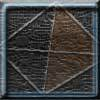### Tetrahedron Faces

##### Age 7 to 11 Challenge Level:

One face of a regular tetrahedron is painted blue and each of the remaining faces are painted using one of the colours red, green or yellow. How many different possibilities are there?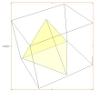### A Mean Tetrahedron

##### Age 11 to 14 Challenge Level:

Can you number the vertices, edges and faces of a tetrahedron so that the number on each edge is the mean of the numbers on the adjacent vertices and the mean of the numbers on the adjacent faces?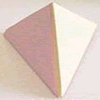### Child's Play

##### Age 7 to 11 Challenge Level:

A toy has a regular tetrahedron, a cube and a base with triangular and square hollows. If you fit a shape into the correct hollow a bell rings. How many times does the bell ring in a complete game?### Triangles to Tetrahedra

##### Age 11 to 14 Challenge Level:

Imagine you have an unlimited number of four types of triangle. How many different tetrahedra can you make?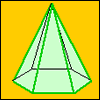### A Chain of Eight Polyhedra

##### Age 7 to 11 Challenge Level:

Can you arrange the shapes in a chain so that each one shares a face (or faces) that are the same shape as the one that follows it?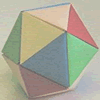### Paper Folding - Models of the Platonic Solids

##### Age 11 to 16

A description of how to make the five Platonic solids out of paper.### Lighting up Time

##### Age 7 to 14 Challenge Level:

A very mathematical light - what can you see?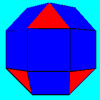### Rhombicubocts

##### Age 11 to 14 Challenge Level:

Each of these solids is made up with 3 squares and a triangle around each vertex. Each has a total of 18 square faces and 8 faces that are equilateral triangles. How many faces, edges and vertices. . . .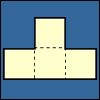### Cut Nets

##### Age 7 to 11 Challenge Level:

Each of the nets of nine solid shapes has been cut into two pieces. Can you see which pieces go together?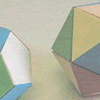### Face Painting

##### Age 7 to 11 Challenge Level:

You want to make each of the 5 Platonic solids and colour the faces so that, in every case, no two faces which meet along an edge have the same colour.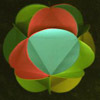### Making Maths: Triangular Ball

##### Age 7 to 11 Challenge Level:

Make a ball from triangles!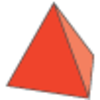### Next Size Up

##### Age 7 to 11 Challenge Level:

The challenge for you is to make a string of six (or more!) graded cubes.### Which Solids Can We Make?

##### Age 11 to 14 Challenge Level:

Interior angles can help us to work out which polygons will tessellate. Can we use similar ideas to predict which polygons combine to create semi-regular solids?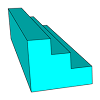### Which Solid?

##### Age 7 to 16 Challenge Level:

This task develops spatial reasoning skills. By framing and asking questions a member of the team has to find out what mathematical object they have chosen.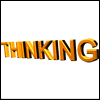### Thinking 3D

##### Age 7 to 14

How can we as teachers begin to introduce 3D ideas to young children? Where do they start? How can we lay the foundations for a later enthusiasm for working in three dimensions?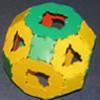### Platonic and Archimedean Solids

##### Age 7 to 16 Challenge Level:

In a recent workshop, students made these solids. Can you think of reasons why I might have grouped the solids in the way I have before taking the pictures?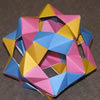### Plaited Origami Polyhedra

##### Age 7 to 16 Challenge Level:

These models have appeared around the Centre for Mathematical Sciences. Perhaps you would like to try to make some similar models of your own.